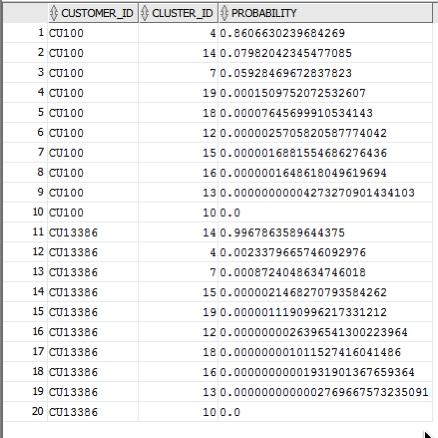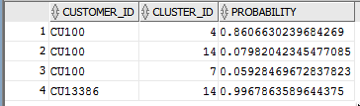### Cluster Sets using SQL with Oracle Data Mining – Part 3

Posted on Updated on

This is the third blog post on my series on examining the Clusters that were predicted by an Oracle Data Mining model. Check out the previous blog posts.

In the previous posts we were able to list the predicted cluster for each record in our data set. This is the cluster that the records belonged to the most. I also mentioned that a record could belong to many clusters.

So how can you list all the clusters that the a record belongs to?

You can use the CLUSTER_SET SQL function. This will list the Cluster Id and a probability measure for each cluster. This function returns a array consisting of the set of all clusters that the record belongs to.

The following example illustrates how to use the CLUSTER_SET function for a particular cluster model.

```SELECT t.customer_id, s.cluster_id, s.probability
FROM   (select customer_id, cluster_set(clus_km_1_37 USING *) as Cluster_Set
from   insur_cust_ltv_sample
WHERE  customer_id in ('CU13386', 'CU100')) T,
TABLE(T.cluster_set) S
order by t.customer_id, s.probability desc;
```

The output from this query will be an ordered data set based on the customer id and then the clusters listed in descending order of probability. The cluster with the highest probability is what would be returned by the CLUSTER_ID function. The output from the above query is shown below.If you would like to see the details of each of the clusters and to examine the differences between these clusters then you will need to use the CLUSTER_DETAILS function (see previous blog post).

You can specify topN and cutoff to limit the number of clusters returned by the function. By default, both topN and cutoff are null and all clusters are returned.

– topN is the N most probable clusters. If multiple clusters share the Nth probability, then the function chooses one of them.

– cutoff is a probability threshold. Only clusters with probability greater than or equal to cutoff are returned. To filter by cutoff only, specify NULL for topN.

You may want to use these individually or combined together if you have a large number of customers. To return up to the N most probable clusters that are greater than or equal to cutoff, specify both topN and cutoff.

The following example illustrates using the topN value to return the top 4 clusters.

```SELECT t.customer_id, s.cluster_id, s.probability
FROM   (select customer_id, cluster_set(clus_km_1_37, 4, null USING *) as Cluster_Set
from   insur_cust_ltv_sample
WHERE  customer_id in ('CU13386', 'CU100')) T,
TABLE(T.cluster_set) S
order by t.customer_id, s.probability desc;
```

and the output from this query shows only 4 clusters displayed for each record.Alternatively you can select the clusters based on a cut off value for the probability. In the following example this is set to 0.05.

```SELECT t.customer_id, s.cluster_id, s.probability
FROM   (select customer_id, cluster_set(clus_km_1_37, NULL, 0.05 USING *) as Cluster_Set
from   insur_cust_ltv_sample
WHERE  customer_id in ('CU13386', 'CU100')) T,
TABLE(T.cluster_set) S
order by t.customer_id, s.probability desc;
```

and the output this time looks a bit different.Finally, yes you can combine these two parameters to work together.

SELECT t.customer_id, s.cluster_id, s.probability
FROM (select customer_id, cluster_set(clus_km_1_37, 2, 0.05 USING *) as Cluster_Set
from insur_cust_ltv_sample
WHERE customer_id in (‘CU13386’, ‘CU100’)) T,
TABLE(T.cluster_set) S
order by t.customer_id, s.probability desc;